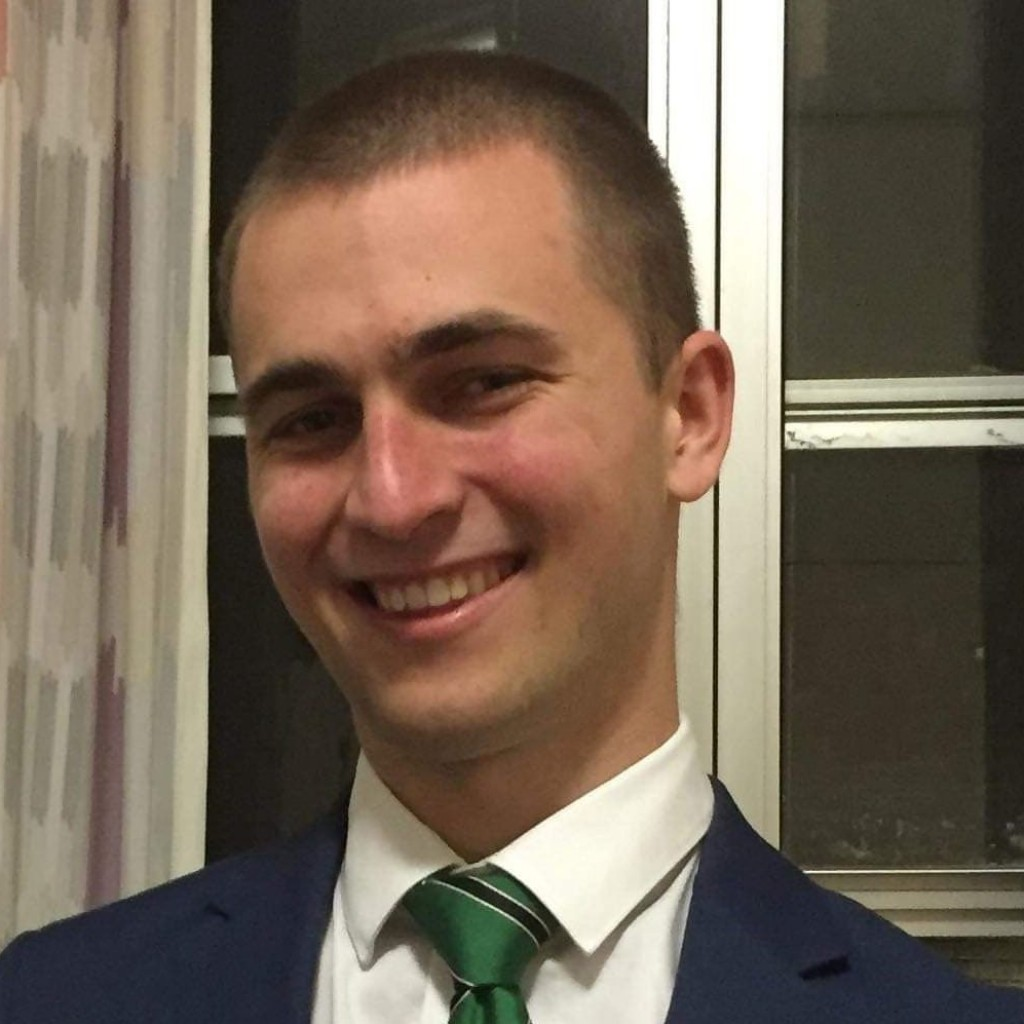Over the course of my project, what I found was most interesting was all the unexpected interconnections and relations that my project had with other topics from all areas of mathematics and science, even from what would seem to be entirely disparate studies. I discuss some of the connections that I found most intriguing here.

The subject of my project was a partial differential equation, a type of equation which is designed to describe things in terms of how they change. This is the part of mathematics which I find most interesting, as the field often has many real-world applications and physical interpretations due to the powerfully descriptive nature of such equations. The particular equation I was to study is named the curve shortening flow, an equation which perhaps unsurprisingly affects curves over time. The actual focus of my project was to look into the properties of this equation so that I could come to understand a famous theorem of Matthew Grayson, but over the course of the project I was incredibly interested to learn that this equation, like so many others within the scope of partial differential equations, not only describes some observable interactions in the real world, but also has applications in computer science and even in some mathematical proofs that I would never have realised was related if not for this project.

The curve shortening flow in two dimensions is just the one-dimensional case of the mean curvature flow, a more general equation that has quite a number of applications, and as such curve shortening flow inherits a number of physical applications from this equation. The mean curvature flow describes the grain boundary of annealing metals, and can be used to model the boundary between two liquids which don’t mix, which in this case means that in a sheet of metal or a film of immiscible liquids, the much simpler curve shortening flow equation can be used to effectively model both of these processes.

Because the effect of curve shortening flow on curves is to effectively smooth them, the equation can be applied in order to smooth out shapes for better analysis by computers, which is particularly useful when it comes to removing noise. Additionally, in image processing, a median filter is a method of removing noise from a picture; and in two colour pictures, the movement of the colour boundaries after repeatedly applying this filter can be modelled by the curve shortening flow. It is incredibly interesting to see the equation that I’m studying crop up in all sorts of unusual scenarios.

For me, perhaps the most fascinating aspect of this equation is its usage in further mathematics. There are several other proofs whose conclusions can be at least partially derived from the properties of curve shortening flow, for example in the theorem of three geodesics. My study of this topic has really driven home the interconnected web that is mathematics, and I can’t wait to see more.

Nicholas Garrett
University of New EnglandView Nicholas Garrett
Profile